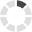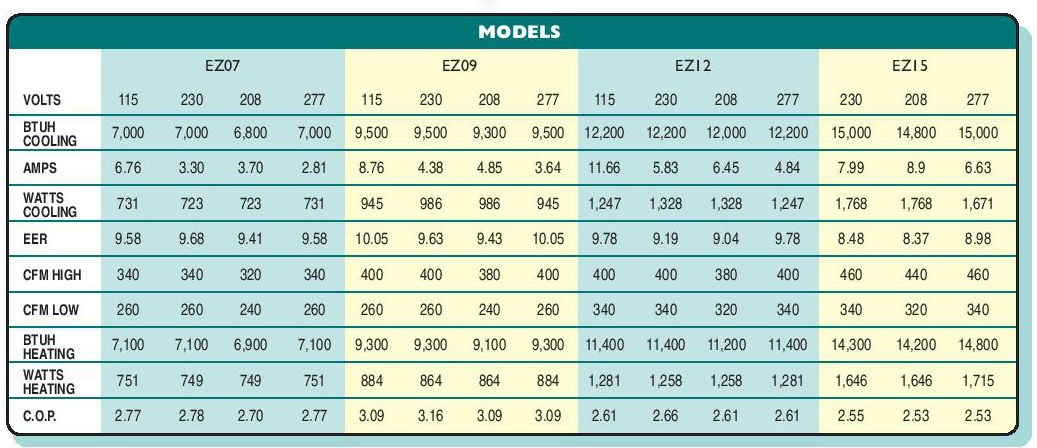### Site InformationLoading... Please wait...

## HVAC News that you don't want to miss.

We constantly publish fresh content about news and products in the HVAC industry. This information is designed to help you, but you'll need to subscribe below!

# Determining the energy effciency of an HVAC system

Posted on

Measuring efficiency for  HVAC systems helps compare the effectiveness of different units in an easy and equitable manner. Depending on the system and application, there are several methods to measure efficiency, providing useful information to help in selection of the HVAC system and providing predicted energy consumption estimates. Among the most common efficiency measurements are Seasonal Energy Efficiency Ratio (SEER) and Energy Efficiency Ratio (EER). Let's take a look at both.

## Seasonal Energy Efficiency Ratio (SEER)

Seasonal Energy Efficieny Ratio measures the total heat removed from the conditioned space during the annual cooling season, expressed in Btu’s, divided by the total electrical energy consumed by the air conditioner or heat pump during the same season, expressed in watt-hours. Rating is only applicable to units under 6 tons (65,000 BTU/h). The minimum SEER rating value established by the Department of Energy (DOE) is 13.

SEER can be used to predict the annual cost of energy will be consumed by the HVAC system using the following formula:

The annual cost of the electric energy = unit size (BTU/h) × hours per year (h) × energy cost (\$/kWh) ÷ (SEER x 1000 BTU/KWh)

Example 1

A residence has an air conditioner with a cooling capacity of 4 tons and an SEER rating of 13. The unit is operated 120 days each year for 8 hours per day (960 hours per year), assume the electric energy cost is \$0.10 per kilowatt-hour. What is the annual cost of the electric energy required to operate the air conditioner?

First, we convert tons of cooling to BTU/h:

(4 tons) × (12,000 BTU/h/ton) = 48,000 Btu/h.

The annual cost of the electric energy is:

(48,000 Btu/h) × (960 h/year) × (\$0.10/kWh) ÷ (13x1000 Btu/KWh) = \$354/year

Example 2

Consider the same conditions as in Example.1 but SEER rating of 20.

The annual cost of the electric energy is:

(48,000 Btu/h) × (960 h/year) × (\$0.10/kWh) ÷ (20x1000 Btu/KWh) = \$230/year

As we can see form the above examples, if we select AC with SEER rating of 20 instead of 13, we would save \$125 annually.

## Energy efficiency ratio (EER)

Energy Efficiency Ratio is the ratio of output cooling energy (BTU) to input electrical energy (Wh) at a given operating point. EER is generally calculated using a 95 °F outside temp and an inside (actually return air) temp of 80 °F and 50% relative humidity. So EER usually used to predict the energy consumption at peak loads.

Typical EER for residential central cooling units = 0.875 × SEER. SEER is a higher value than EER for the same equipment.

Example 3

A residence has an air conditioner with a cooling capacity of 4 tons and an EER rating of 11. The unit is operated at peak load at 95 °F outside temp and an inside temp of 80 °F, assume the electric energy cost is \$0.10 per kilowatt-hour. What is the cost of the electric energy required to operate the air conditioner during peak time (assuming the unit operate at peak loads 300 hours)?

First, we convert tons of cooling to BTU/h:

(4 tons) × (12,000 BTU/h/ton) = 48,000 Btu/h.

EER = Unit size (BTU/h) ÷ Electrical input (Wh)

Electrical Input = Unit size (BTU/h) ÷ EER = 48,000 ÷ 11 = 4,364 Wh = 4.364 KWh

Total electrical input during peak hours = 4.364 x 300 = 1,309 KWh

The cost of the electric energy = Energy cost (\$/KWh) x Total Electrical Input (KWh)

= 0.1 x 1,309 = \$130.9

Example 4

Same as above but EER value is 15

Electrical Input = Unit size (BTU/h) ÷ EER = 48,000 ÷ 15 = 3,200 Wh = 3.2 KWh

Total electrical input during peak hours = 3.2 x 300 = 960 KWh

The cost of the electric energy = Energy cost (\$/KWh) x Total Electrical Input (KWh)

= 0.1 x 960 = \$96

As we can see form the above examples, if we select AC with EER rating of 15 instead of 11, at the same conditions we would save \$35 during peak hours.

Example 5.  PTAC unit which is a direct replacement of McQuay K model has the following data sheet. What is the cost of the electric energy required to operate the unit model E12EER = Unit size (BTU/h) ÷ Electrical input (Wh), so Electrical input (Wh) = Unit size (BTU/h) ÷ EER

In cooling mode, at voltage 115, cooling capacity = 12,200 BTU/h, EER = 9.78

Electrical input (Wh) = 12,200/9.78=1247.4 =1.25 kWh, assuming \$0.10 per kilowatt-hour and the unit is running on peak load for 800 hours. Then the cost of electricity would be = 1.25 kWh x \$0.10 x 800 hr = \$100